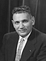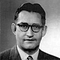# KL Divergence Demystified

What does KL stand for? Is it a distance measure? What does it mean to measure the similarity of two probability distributions?Source: https://www.freeimages.com/photo/blue-strings-4-1542735

If you want to intuitively understand what the KL divergence is, you are in the right place, I’ll demystify the KL divergence for you.

As I’m going to explain the KL divergence from the information theory point of view, it is required to know the entropy and the cross-entropy concepts to fully apprehend this article. If you are not familiar with them, you may want to read the following two articles: one for the entropy and the other for the cross-entropy.

If you are ready, read on.

# What does KL stand for?

KL in the KL divergence stands for Kullback-Leibler which represents the following two people:Solomon Kullback (Source: Wikipedia)Richard A. Leibler (Source: NSA)

They introduced the concept of the KL divergence in 1951 (Wikipedia).

# What is the KL divergence?

The KL divergence tells us how well the probability distribution Q approximates the probability distribution P by calculating the cross-entropy minus the entropy.

As a reminder, I put the cross-entropy and the entropy formula as below:

The KL divergence can also be expressed in the expectation form as follows:

The expectation formula can be expressed in the discrete summation form or in the continuous integration form:

So, what does the KL divergence measure? It measures the similarity (or dissimilarity) between two probability distributions.

If so, is the KL divergence a distance measure?

To answer this question, let’s see a few more characteristics of the KL divergence.

# The KL divergence is non-negative

The KL divergence is non-negative. An intuitive proof is that:

• if P=Q, the KL divergence is zero as:
• if P≠Q, the KL divergence is positive because the entropy is the minimum average lossless encoding size.

So, the KL divergence is a non-negative value that indicates how close two probability distributions are.

It does sound like a distance measure, doesn’t it? But it is not.

# The KL divergence is asymmetric

The KL divergence is not symmetric:

It can be deduced from the fact that the cross-entropy itself is asymmetric. The cross-entropy H(P, Q) uses the probability distribution P to calculate the expectation. The cross-entropy H(Q, P) uses the probability distribution Q to calculate the expectation.

So, the KL divergence cannot be a distance measure as a distance measure should be symmetric.

This asymmetric nature of the KL divergence is a crucial aspect. Let’s look at two examples to understand it intuitively.

Suppose we have a probability distribution P which looks like below:

Now, we want to approximate it with a normal distribution Q as below:

The KL divergence is the measure of inefficiency in using the probability distribution Q to approximate the true probability distribution P.

If we swap P and Q, it means that we use the probability distribution P to approximate the normal distribution Q, and it’d look like below:

Both cases measure the similarity between P and Q, but the result could be entirely different, and they are both useful.

# Modeling a true distribution

By approximating a probability distribution with a well-known distribution like the normal distribution, binomial distribution, etc., we are modeling the true distribution with a known one.

This is when we are using the below formula:

Calculating the KL divergence, we can find the model (the distribution and the parameters) that fits the true distribution well.

# Variational Auto-encoder

An example of using the below formula is the variational auto-encoder.

I will lightly touch on this topic here as it requires a lot more to explain for people who are not familiar with the variational auto-encoder.

The KL divergence is used to force the distribution of latent variables to be a normal distribution so that we can sample latent variables from the normal distribution. As such, the KL divergence is included in the loss function to improve the similarity between the distribution of latent variables and the normal distribution.

I may write more about the variational auto-encoder in future if people are interested in. Please let me know in the comment section.

# A few minor mathy points

The term p log p becomes zero when p goes to zero.

When p>0 but q=0, it is defined as infinity.

A more rigor proof of the KL divergence being non-negative is as follows:

Since -log is a convex function, we can apply Jensen’s inequality:

# Likelihood Ratio

There is another way to describe the KL divergence from a probabilistic perspective in that the following likelihood ratio is used.

If you are interested in this approach, I recommend the article by Marko Cotra (the link in the references section below).

~ ~ ~ ~ ~ ~ ~ ~ ~ ~ ~ ~ ~ ~ ~ ~ ~ ~ ~ ~ ~ ~ ~ ~ ~ ~ ~ ~ ~ ~ ~

That is all for now. I hope this article is useful to you.

# Related Articles

Demystifying Cross-Entropy

Demystifying Entropy

# References

Kullback Leibler divergence (Wikipedia)

https://en.wikipedia.org/wiki/Kullback%E2%80%93Leibler_divergence

CS412 Fall 2008. Introduction to Data Warehousing and Data Mining

Jiawei Han

http://hanj.cs.illinois.edu/cs412/bk3/KL-divergence.pdf

ECE 830 Fall 2011 Statistical Signal Processing

Robert Nowak

http://nowak.ece.wisc.edu/ece830/ece830_fall11_lecture7.pdf

Making sense of the Kullback–Leibler (KL) Divergence

Marko Cotra

https://medium.com/@cotra.marko/making-sense-of-the-kullback-leibler-kl-divergence-b0d57ee10e0a

A Short Introduction to Entropy, Cross-Entropy, and KL-Divergence

Aurélien Géron

https://www.youtube.com/watch?v=ErfnhcEV1O8

## More from Naoki

https://twitter.com/naokishibuya